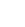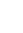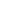Co-authored by

• Don Welch, Director of Engineering and Business Development
• Cory Bufi, Software Engineer
• Isaac Abbott,Product Innovation Engineer – PBS Group

Today’s electronic designs often call for reasonably fast and precise positioning of miniature components for process deposition elements such as ink jet nozzles, 3D printing elements etc. And as process control moves from mm positioning to micron to sub-micron positioning it becomes necessary to monitor and control positioning actuators such as piezo flexure stage and voice coil motors. Open loop control of the positioning stage may have been adequate in non-precision applications but piezo electric stages and voice coil motors don’t have a super accurate open loop linear response. Open loop control voltage or motor current input is simply not precise enough for these applications. Feedback is necessary for accuracy using capacitance sensors, strain gauges or laser interferometers.

Capacitance sensors are far less expensive than laser interferometers but have the stability and precision of laser interferometers. They are also more accurate than strain gauges and other contact displacement gauges such as LVDT. Capacitance sensors also don’t touch the piezo stage or voice coil motor so there are no mechanical loading effects. Digital feedback control is gaining popularity as the desired method rather than older all-analog techniques.

This application note describes how to use a MTI digital displacement sensor as a position sensor in the feedback control system with a precision piezo electric positioning stage. It describes how to implement a complete digital closed loop control using an example with a Piezo System Jena stage. It shows the components needed for a real world implementation and lists some of the latency and delay parameters encountered. It was not our intent to make the closed loop response as fast as possible but rather have a reasonably fast closed loop response and to determine the latency of the MTI Digital capacitance sensor.

Although analog capacitance sensors have been used for decades only recently have they been available as digital sensors. A typical closed loop control system diagram is shown in Fig 1.Figure 1: Typical closed loop control system

The process variable (motion stage or motor) can be an anything from a stepper motor to linear slide. For precision applications this often becomes a piezo flexure stage or voice coil motor (VCM). The amplifier drives the motor and can be a servo amplifier or high voltage amplifier for a piezo electric stage.

In this case the process (motion stage) feedback is an MTI capacitance probe connected to a Digital Accumeasure Amplifier that has Ethernet output. In our example the programmable motion controller will be an Intel Atom CPU with real time operating system software (RTOS) . RTOS was chosen to avoid the huge latency found in a typical Windows environment.

The motion controller accepts a commanded position, which then uses position feedback to ensure the motor stage goes to exactly where it’s commanded to within sub-micron precision.

In our application we will use the following block diagram (Fig 2.)Figure 2 – Control loop test configuration

## Controller Overview

The loop controller shown in Figure 2is a real time operating system (RTOS) CPU in. Its primary function is to keep the measured process variable (PV) as close as possible to the command position set point (SP) at all times. When either a change in set point or process disturbance occurs, the controller must detect such changes, perform calculations based on these changes, and then issue a control output action (CO) that will bring the process and the current set point into correspondence.Figure 3 – Traditional PID control loop

The controller uses a traditional PID control loop (Figure 3). All inputs and outputs are digital, with the latter processed through a digital-to-analog converter. Without getting into the detail, the runtime code is modeled in Figure 4. Statements 1 and 2 correspond to the controller input (i.e., set point and process measurement updates), while statement 8 corresponds to the controller output (i.e., a function of the input and PID values). The controller uses Statements 3 through 7 to implement the PID controller function. Collectively, statements 1 through 8 constitute the control loop, and the time it takes to execute the control loop, that is, one control loop iteration, is referred to as the loop cycle processing time, T.Figure 4 – High-level description of control algorithm being run by the RTOS control processor

While a specific value for T is application dependent, in general it is desired that T be periodic to within some tolerance.  Any variation in T from one iteration to another is known as jitter. The major sources of jitter are irregularities in the time needed to execute each statement of the control algorithm as well as the delays in receiving data over the Ethernet.

The runtime operation of our control algorithm relies on three tasks as shown in Figure 5.  The IP task is responsible for Ethernet communications with the Digital Accumeasure, while the Dacc task is responsible for making the PV data available to the Motion Task.  The Motion Task performs the calculations that determine the appropriate control output for each iteration.  As we will show in the next section, the control loop cycle processing time realized for this controller was 500.Figure 5 -Main controller task architecture

The next step is to determine the constants Kp,  Ki and Kd. In order to do this we will use the first order plus dead time model (FOPDT) to determine the open loop response of the Piezo stage + Piezo amplifier and the latency of the Digital Accumeasure capacitance probe along with the latency of the CPU loop and the dead time in the Ethernet communications.

## Determining PID Parameters

To derive the PID coefficients in (Fig 3 & 4), we first measure the FOPDT parameters θ,κ, and τ from an open-loop step response. The values obtained are then input to a set of correlation functions that return the proportional, integral and derivative valuesKp,  Ki and Kd, respectively. The PID coefficients are further refined through several closed-loop tests aimed at moderate to aggressive control.

### Open-Loop Test

The open-loop test consists of a single 30 micron step change that assumes a nominal 25 micron operating point. We perform this test for loop time T=200μs and then again at T=500μs.  We do this for two reasons. The first reason is to determine how fast the loop time can be executed without introducing too much jitter. The second reason is to compare the time constant and dead time measured at the two loop rates.  Higher loop rates correspond to higher sample rates, which in turn improve measurement accuracy, while lower loop rates allow more time to execute the loop statements, thus realizing lower jitter and more consistent response times.

The step input and corresponding system response for the two single step tests are shown in Figure 6 and Figure 7. The sample points are highlighted in the middle figures to emphasize the difference in resolution: approximately 12 points are available to compute the time constant from the higher rate data, while less than half that number are available for computing the time constant using the lower rate data.Figure 6: Open-loop single-step test at 200us loop time, SP is the command setpoint, PV is the measured value of the stage position , CO is the controller output

Conversely, as expected, jitter performance is better at the lower loop rate, as shown by the bottom figures of Figure 6 and Figure 7.

Table 1 compares the graphically computed FOPDT model parameters dead time , gain , and time constant  obtained from Fig 6 and Fig 7.  The results show strong agreement between the two loop rates forand.  The difference of roughly  for the time constant is on the order of the difference in loop times and is to be expected since this time is approximately the difference in -axis resolution between the two tests.

Using the FOPDT parameters calculated in Table 1, the PID values are determined from the following correlations:The derived PI coefficients are shown in Table 2.Table 2: Derived PI coefficients

Figure 8 compares the closed-loop performance for the same test profile used in Figure 6 and Figure 7. All closed-loop tests were performed was T = 500.  The top figure shows the result using moderate tuning values for T = 200in Table 2.  In this case, we have used a PI controller tuned for one loop rate (T = 200.) and applied it to another, slower rate (T = 500).  The middle and bottom figures show the results for moderate and aggressive tuning values, respectively, for T = 500.

Comparing the results shown in Figure 8, the middle achieves a faster response time than the top, while the response time in the bottom is faster than the middleFigure 6: Open-loop single-step test at 200us loop time, SP is the command setpoint , PV is the measured value of the stage position , CO is the controller outputFigure 7: Open-loop single-step test at 500us controller loop time

### Closed-Loop PI Controller

Figure 8 presents the closed-loop performance for three PI controller implementations using values from Table 2. All closed-loop tests were performed using the step profile that was used in Figure 6 and Figure 7, and with T = 500. The top figure shows the result using moderate tuning values for T = 200in Table 2. The middle and bottom figures show the results for moderate and aggressive tuning values forT = 500in Table 2.

Comparing the results shown in Figure 8, the top figure shows a slower response time than the response time in the middle figure by approximately a factor of three, even though both are considered moderate tunings. Unlike the middle figure, the top figure represents the case in which the PI controller was tested at a slower loop rate than the rate at which it was tuned (i.e.  500,  versus 200). Clearly the loop rate is an important consideration when tuning a PI controller.

The middle and bottom figures represent moderate and aggressive tunings, respectively. The response times reflect these tuning strategies, with aggressive tuning realizing a speedup by nearly a factor of four 4 over moderate tuning. Figure 9 presents these performance differences in greater detail.Figure 8 – Closed Loop TestFigure 9: Closed-loop moderate tuning (left), aggressive tuning (right)

### Closed-Loop PID Controller

Aggressive tuning as shown in the Figure 9 offers faster response time, but it is not without overshoot and some oscillation.  In this section we present the results of a tuning strategy that lies between the moderate and aggressive tunings we saw above. In doing so, we introduce the derivative term, Kd, to our controller design.

A derivative term in PID controllers is known to have sensitivity to noise, but it can also help decrease overshoot and settling time.  Figure 10 shows the PID performance with Kp = 0.006, Ki = 0.005 and Kd = 0.004.  The settling time of this controller is ~50ms, which is faster than the moderate tuning by about a factor of 2.5, and slower by less than twice the settling time of the aggressive tuning, but is also free of oscillation.Fig 10 Critically damped loop response.  The closed loop response of our application is about 50 ms.

Below are what the individual system components look likeThis is the Piezo Stage made by Piezo System  Jena.  It is the PX-100 and has a full range motion of 100um . The stage has an MTI ASP-500M-ILA probe imbedded in it to measure the motion.The driver for the Piezo stage is a high voltage amplifier from Piezo System Jena  it amplifies the 0-10V from the control CPU to 0-100V for the piezo stageThe processor is an Advantek PCM-3365. It has an Intel Atom CPU.  It has Ethernet and a PC-104 stack interface.Diamond systems Analog out board

This is the Diamond systems Analog out board it stacks connects with the controller CPU and used to send a 0-10 volt signal  to the Piezo driver

Digital Accumeasure### Summary

MTI’s Digital Accumeasure makes an effective digital position control sensor. Since the output of the Digital Accumeasure is Ethernet it can be located far from the controller and multiple sensors can be networked with no signal degradation as long as the positions can be read within the controller’s loop control cycle.  In the image below Fig 11 we break out the latency of the MTI Digital Accumeasure (DACC) as a single element.  In the scope position we see the commanded position signal (yellow) and the response of the capacitance probe carrier (blue sinusoidal signal). We measured to the carrier as the carrier represents the Capacitance probe response without actually looking at the gap capacitance because this is impossible without affecting the measurement.  The violet signal is representative of the DACC’s digital output. The violet signal is actually the DACC analog output which is almost instantaneous with the DACC Digital output but lacks the latency of the Ethernet IP. We can see the latency of the DACC is on the order of 2.14 ms.Figure 11

Next we look at the output response of the Digital Accumeasure (Y to PV) . With the filter set to the highest frequency the DACC response (latency ) is about 2.14 ms.

So the DACC latency is only a small portion of the overall loop response (2.14 ms out of 50ms total ) . The total 50 ms closed loop response is the cumulative effect or sum of the control loop timing cycle (500us), the Piezo stage (~ 10 ms), the Digital Accumeasure latency (2.14ms) , the Ethernet communications latency (~ 800us per packet) and the integration effect of the PID loop which takes multiple cycles to stabilize. Remember a control loop cycle in this application is 500us. To decrease the loop response time it’s possible to decrease the controller cycle and also use a faster piezo stage driver amplifier.  Additionally it’s also possible to improve the PID tuning for a faster settling time.

Nov, 3, 2022